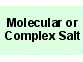Copper(I) cyanide
CuCN
Cuprous cyanideC 1 Cu 1 N 1

Real, long lived, electronically neutral reagent chemical.

Gram formula weight (molecular mass) = 89.563
Melting point = 474 °C
Density = 2.9 g/cm3
Purity = 99 %
Solubility in water = 2.600e-4 g/100mL
Solubility in organics = insoluble

 Wikipedia Page:Copper(I) cyanide
is defined with respect to the entities below:
The entities below are defined with respect to:
Copper(I) cyanide
 Molecular ionic material (generic)Type 23 Lewis acid/base complex (generic)chemical compound molecule metal molecular science reaction mechanism ionic material acid base geometry reactivity synthesis science knowledge chemistry Lewis